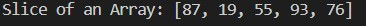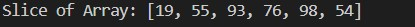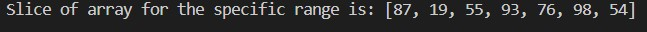# Slice an Array in Java

## Array Slicing in Java

Array slicing is a Java technique for extracting a subarray from a more extensive array.

Assume that `k[]` is an array. It has 7 components, numbered `k` through `k`.

``````k[] = {5, 6, 7, 8, 9, 10, 11}
``````

Now, we’re looking for a slice of the array index between `k` and `k`. The startIndex is `k`, while the endIndex is `k`.

As a result, we obtain the sliced array below:

``````k[] = {7, 8, 9, 10}
``````

In the following sections, we’ll learn how to locate an array slice in Java.

## Slice an Array by Duplicating Elements in Java

It’s a built-in way to acquire a slice of an array. The start and end indexes of the provided array are initially determined in this procedure.

After that, we generate a size-independent empty array (sliced array) (endIndex - startIndex). Copy the items (from startIndex) from the provided array to the sliced array, and lastly, print the sliced array.

Let’s use the way described above in a Java program to acquire a sliced array of the supplied array. We’ll utilize an array of primitive kinds in this application.

``````import java.util.Arrays;

public class Copy {

public static int[] getSlice(int[] arr, int stIndx, int enIndx) {

int[] sclicedArr = new int[enIndx - stIndx];

for (int i = 0; i < sclicedArr.length; i++) {
sclicedArr[i] = arr[stIndx + i];
}

return sclicedArr;
}

public static void main(String args[]) {

int[] arr = { 20, 65, 87, 19, 55, 93, 76, 98, 54, 21 };

int stIndx = 2, enIndx = 6;

int[] sclicedArr = getSlice(arr, stIndx, enIndx + 1);

System.out.println("Slice of an Array: " + Arrays.toString(sclicedArr));
}
}
``````

To run the code, save the program as a Java file and run it in your Java IDE.

Output:## Slice an Array Using the `copyOfRange()` Method in Java

The Java Array class contains the `copyOfRange()` method. It replicates the array’s stated range to a newly formed array (slice array) and returns the newly produced array with the original array’s specified range.

Slicing an array requires `O(n)` time and `O(n)` space to store the elements, where `n` is the number of elements in the resultant array.

Syntax:

``````public static int[] copyOfRange(int[] original, int from, int to)
``````

The following 3 parameters are parsed by the method:

• `original`: It is an array with a slice that must be found.
• `from`: It is the index for the first page. It must be in the range of 0 to the array’s length.
• `to`: It is the last index.

The following exceptions are thrown:

If the start index is less than 0 or higher than the length of the original array, an `ArrayIndexOutOfBoundsException` will be thrown. If the start index is more significant than the end index, an `IllegalArgumentException` will be thrown.

If the specified array is null, a `NullPointerException` will be thrown.

``````import java.util.Arrays;

public class Copy1 {

public static int[] slice(int[] arr, int stIndx, int enIndx) {

int[] sclicedArr = Arrays.copyOfRange(arr, stIndx, enIndx);

return sclicedArr;
}

public static void main(String args[]) {

int[] arr = { 20, 65, 87, 19, 55, 93, 76, 98, 54, 21 };
int stIndx = 3, enIndx = 8;

int[] sliceArray = slice(arr, stIndx, enIndx + 1);

System.out.println("Slice of Array: " + Arrays.toString(sliceArray));
}
}
``````

To run the code, save the program as a Java file and run it in your Java IDE.

Output:## Slice an Array Using the Java 8 Stream

We may use the Java 8 Stream to discover the slice of an array by following the instructions below.

• ##### Convert the mapped array to an array using the `toArray()` function.
``````import java.util.Arrays;
import java.util.stream.IntStream;

public class NewClice {

public static int[] findSlice(int[] arr, int stIndx, int enIndx) {

int[] slice_arr = IntStream.range(stIndx, enIndx).map(i -> arr[i]).toArray();

return slice_arr;
}

public static void main(String args[]) {

int[] arr = { 20, 65, 87, 19, 55, 93, 76, 98, 54, 21, 657 };
int stIndx = 2, enIndx = 8;

int[] slice_arr = findSlice(arr, stIndx, enIndx + 1);

System.out.println("Slice of array for the specific range is: " + Arrays.toString(slice_arr));
}
}
``````

To run the code, save the program as a Java file and run it in your Java IDE.